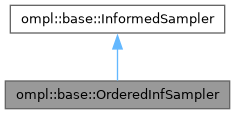ompl::base::OrderedInfSampler Class Reference

An informed sampler wrapper that generates m samples and then returns them in order of the heuristic. More...

#include <ompl/base/samplers/informed/OrderedInfSampler.h>

Inheritance diagram for ompl::base::OrderedInfSampler:[legend]

## Public Member Functions

OrderedInfSampler (const InformedSamplerPtr &infSamplerPtr, unsigned int batchSize)
Construct an ordering wrapper around the provided informed sampler.

bool sampleUniform (State *statePtr, const Cost &maxCost) override
Sample uniformly in the subset of the state space whose heuristic solution estimates are less than the provided cost, i.e. in the interval [0, maxCost). Returns false if such a state was not found in the specified number of iterations.

bool sampleUniform (State *statePtr, const Cost &minCost, const Cost &maxCost) override
Sample uniformly in the subset of the state space whose heuristic solution estimates are between the provided costs, [minCost, maxCost). Returns false if such a state was not found in the specified number of iterations.

bool hasInformedMeasure () const override
Whether the wrapped sampler can provide a measure of the informed subset.

double getInformedMeasure (const Cost &currentCost) const override
The measure of the subset of the state space defined by the current solution cost that is being searched. Passes through to the wrapper sampler.Public Member Functions inherited from ompl::base::InformedSampler
InformedSampler (const InformedSampler &)=delete

InformedSampleroperator= (const InformedSampler &)=delete

InformedSampler (const ProblemDefinitionPtr &probDefn, unsigned int maxNumberCalls)
Construct a sampler that only generates states with a heuristic solution estimate that is less than the cost of the current solution. Requires a function pointer to a method to query the cost of the current solution. If iteration is required, only maxNumberCalls are attempted, to assure that the function returns.

virtual double getInformedMeasure (const Cost &minCost, const Cost &maxCost) const
The measure of the subset of the state space defined by the current solution cost that is being searched. Does not consider problem boundaries but returns the measure of the entire space if no solution has been found or if a closed form expression for the measure does not exist. By default calls the 1-argument overloaded version with the min and max costs and subtracts the differences; however, there may be more efficient ways to do this for some cost functions.

virtual Cost heuristicSolnCost (const State *statePtr) const
A helper function to calculate the heuristic estimate of the solution cost for a given state using the optimization objective stored in the problem definition. More...

ProblemDefinitionPtr getProblemDefn () const

unsigned int getMaxNumberOfIters () constProtected Attributes inherited from ompl::base::InformedSampler
ProblemDefinitionPtr probDefn_
A copy of the problem definition.

OptimizationObjectivePtr opt_
A copy of the optimization objective.

StateSpacePtr space_
A copy of the state space.

unsigned int numIters_
The number of iterations I'm allowed to attempt.

## Detailed Description

An informed sampler wrapper that generates m samples and then returns them in order of the heuristic.

Definition at line 56 of file OrderedInfSampler.h.

The documentation for this class was generated from the following files: